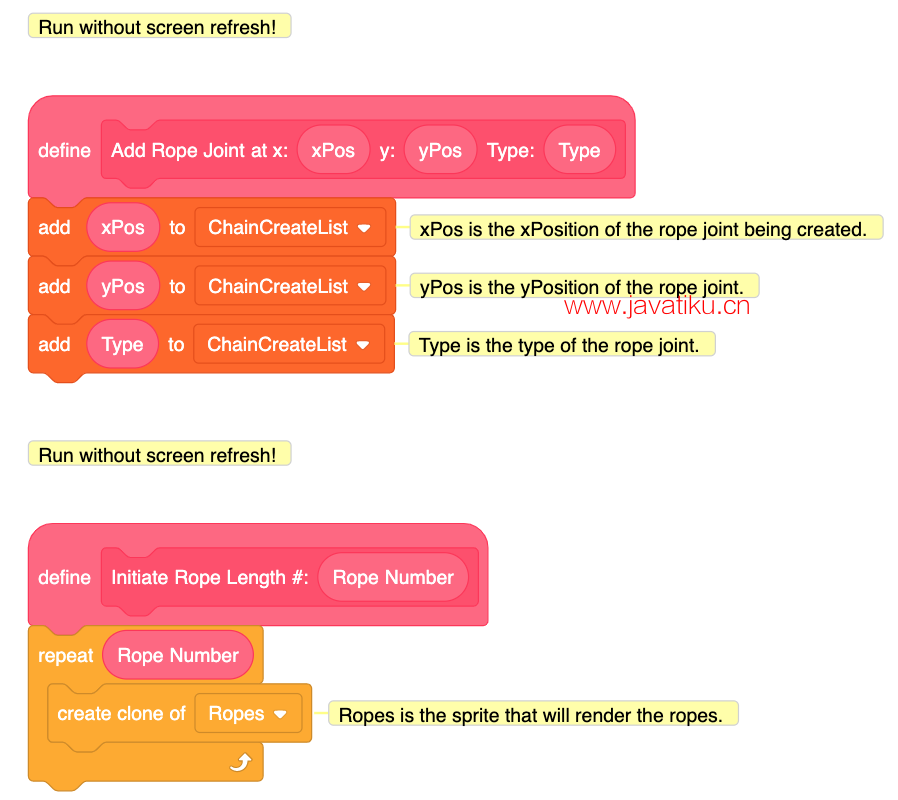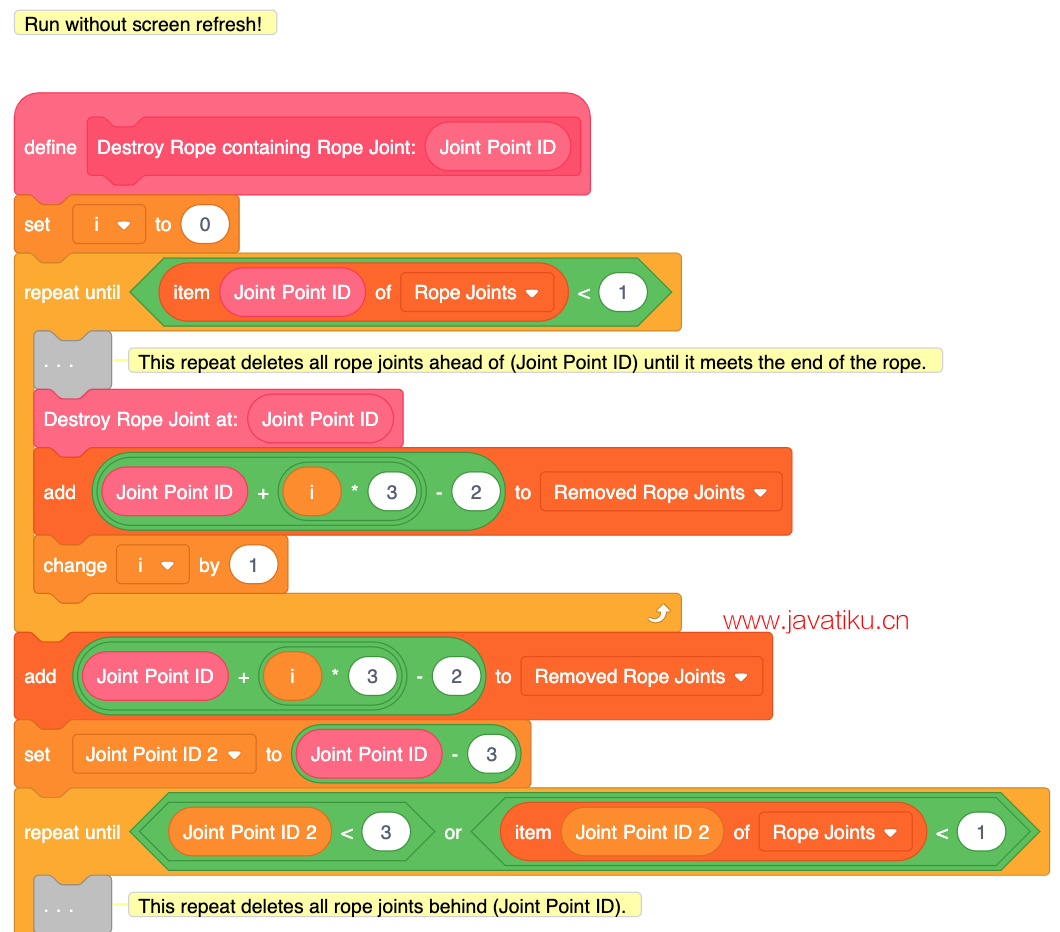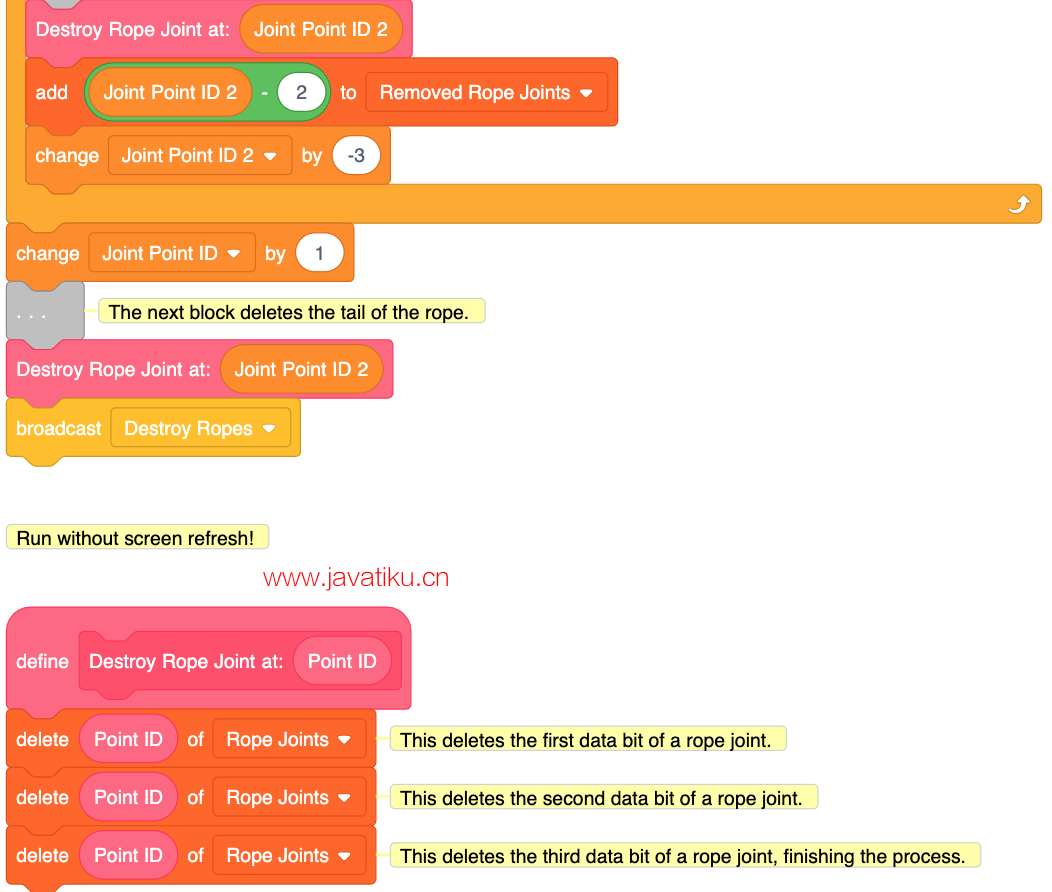# Scratch编程教程-绳索物理：方法3• X速度
• Y速度

• 质量
• 阻尼
• 刚度
• 重力

• X力
• Y力
• 真实的X力
• 真实的Y力
• 制作的绳索关节数量
• 关节ID

• 绳索关节

## 一些背景知识沿y轴的力= y2-y1

X力=（x2-x1）刚度

Y力=（y2-y1）刚度

X力=（（x2-x1）刚度）/质量

Y力=（（y2-y1）刚度）/质量

X速度=（X速度+X力）* 阻尼

Y速度=（Y速度+Y力）* 阻尼

## 编程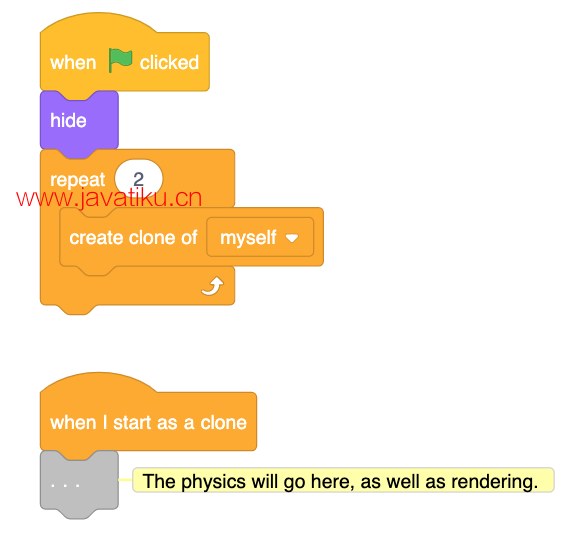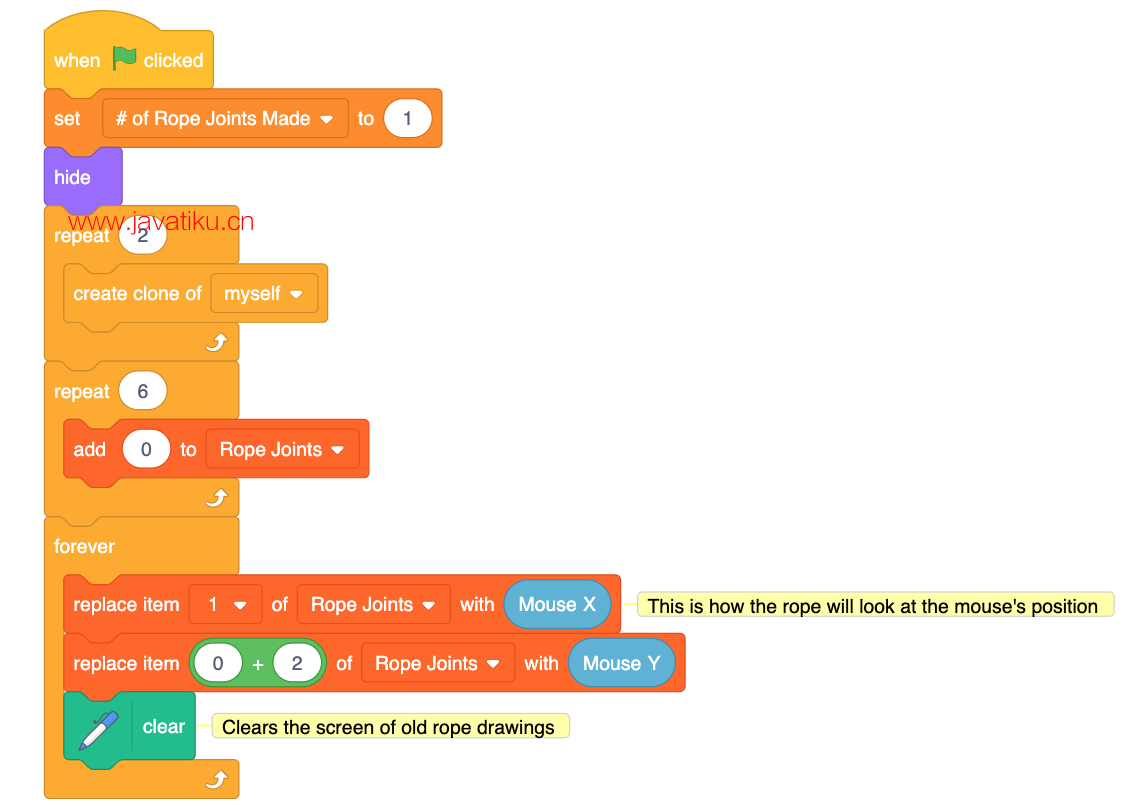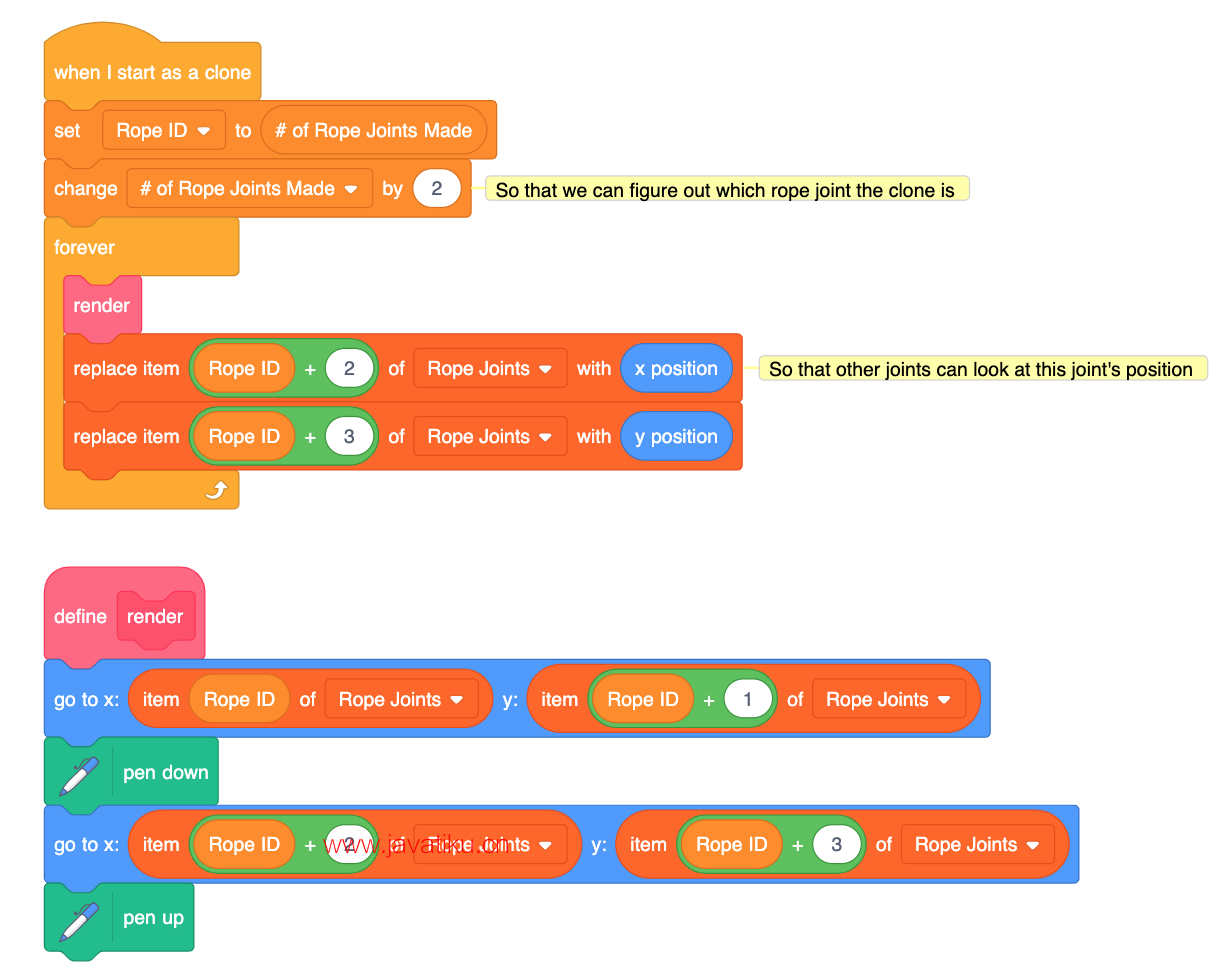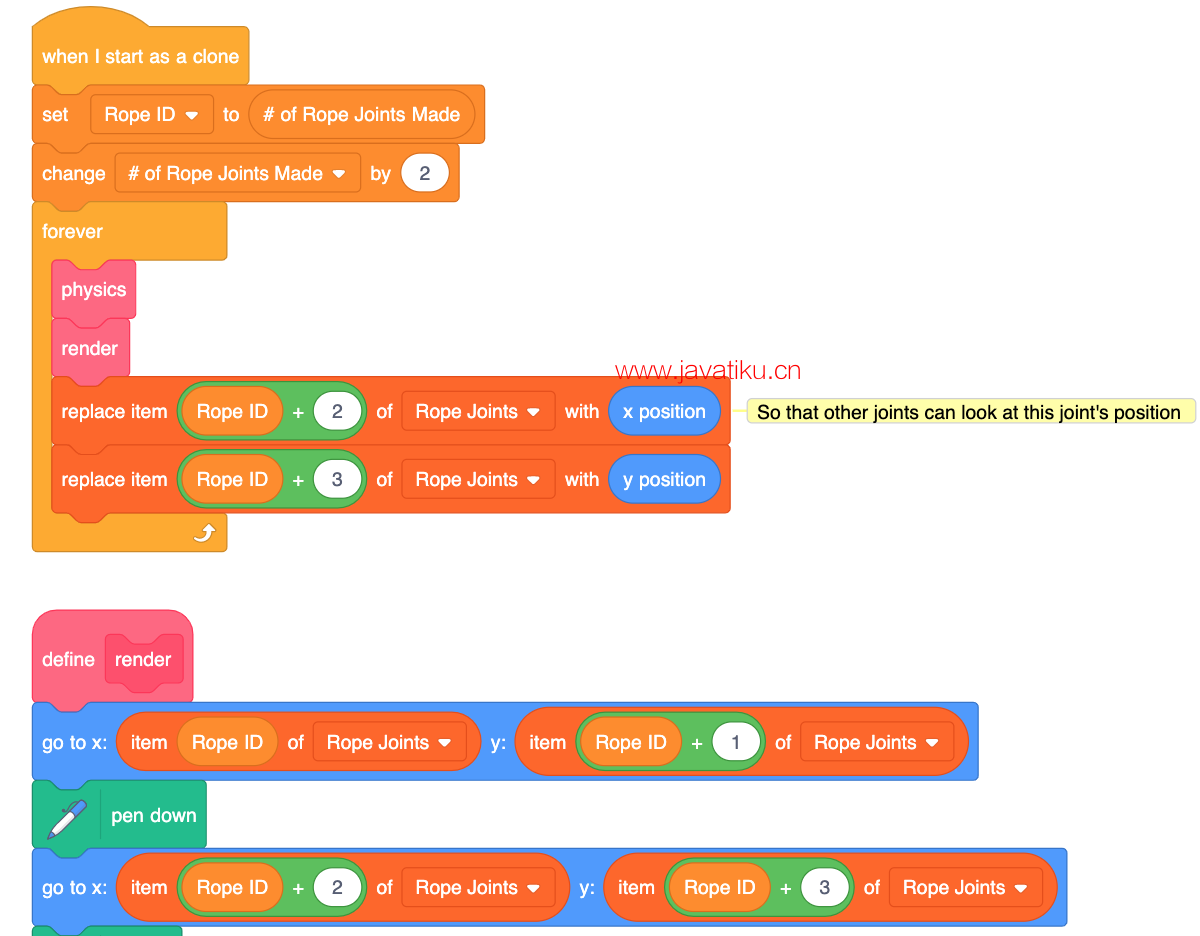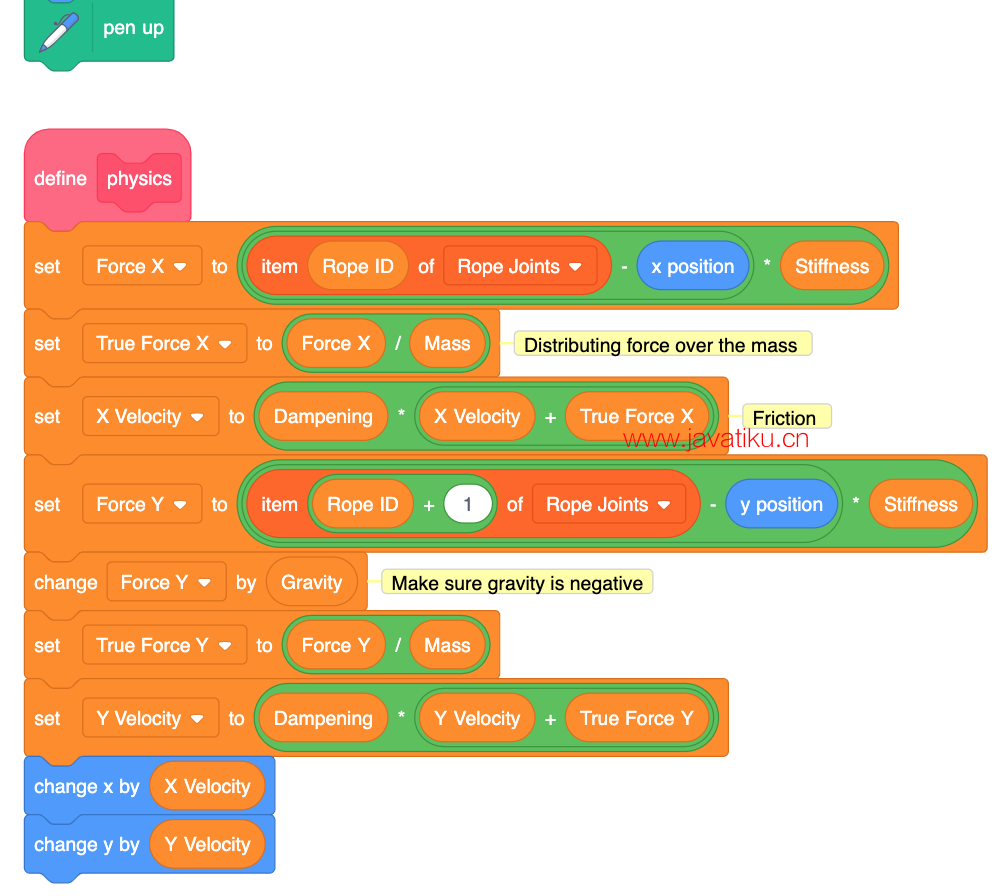## 最终产品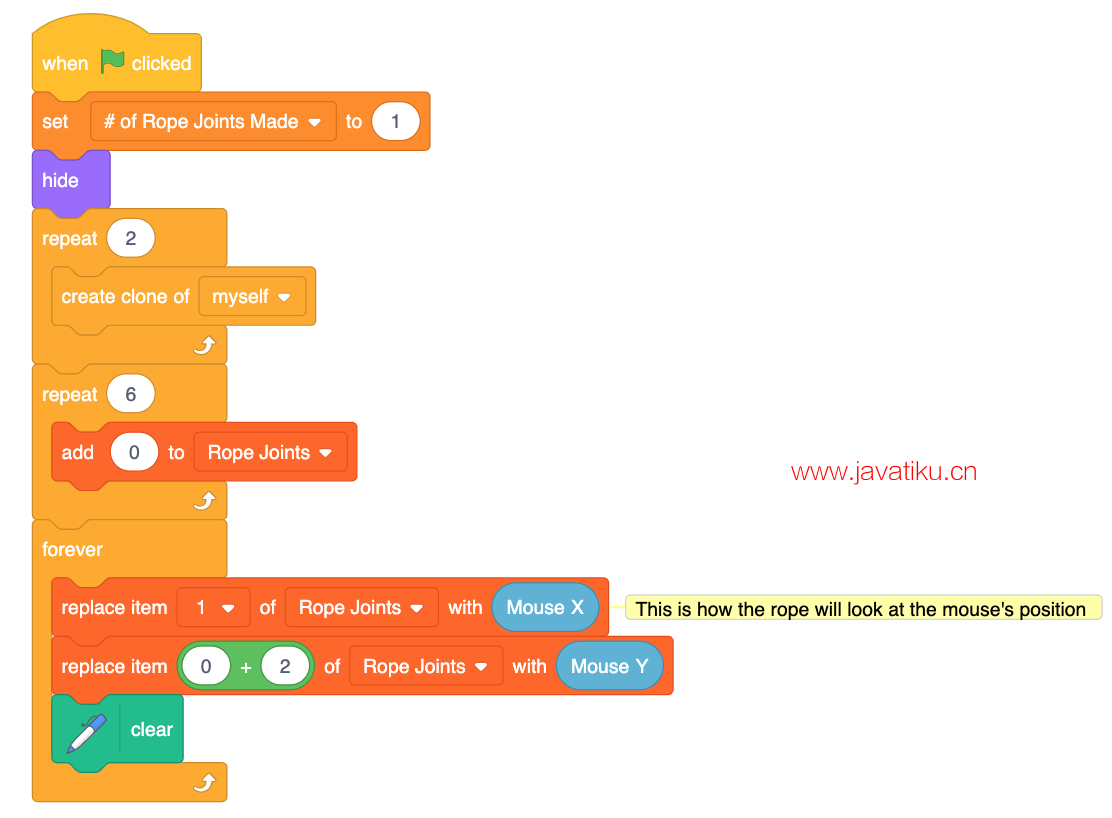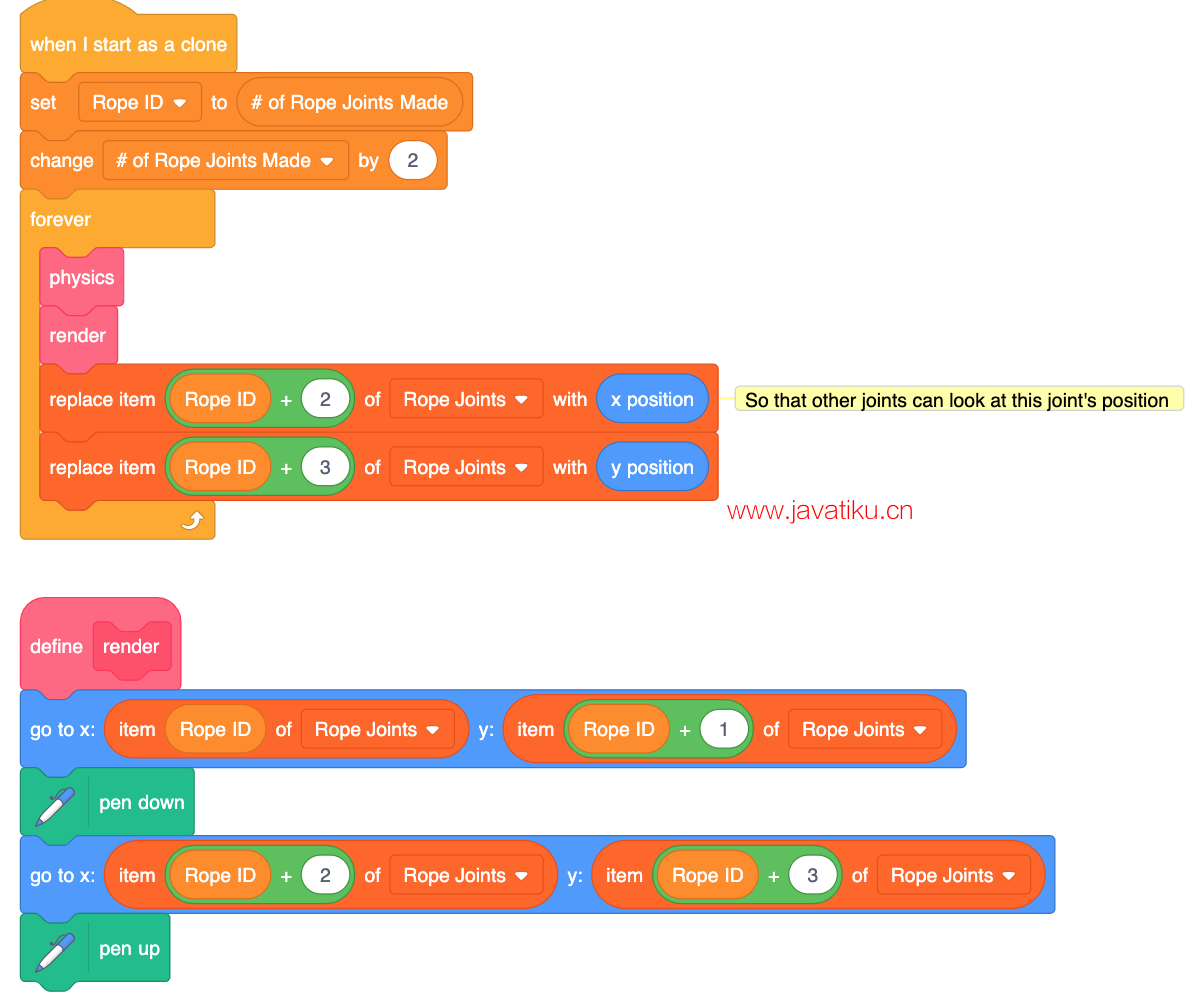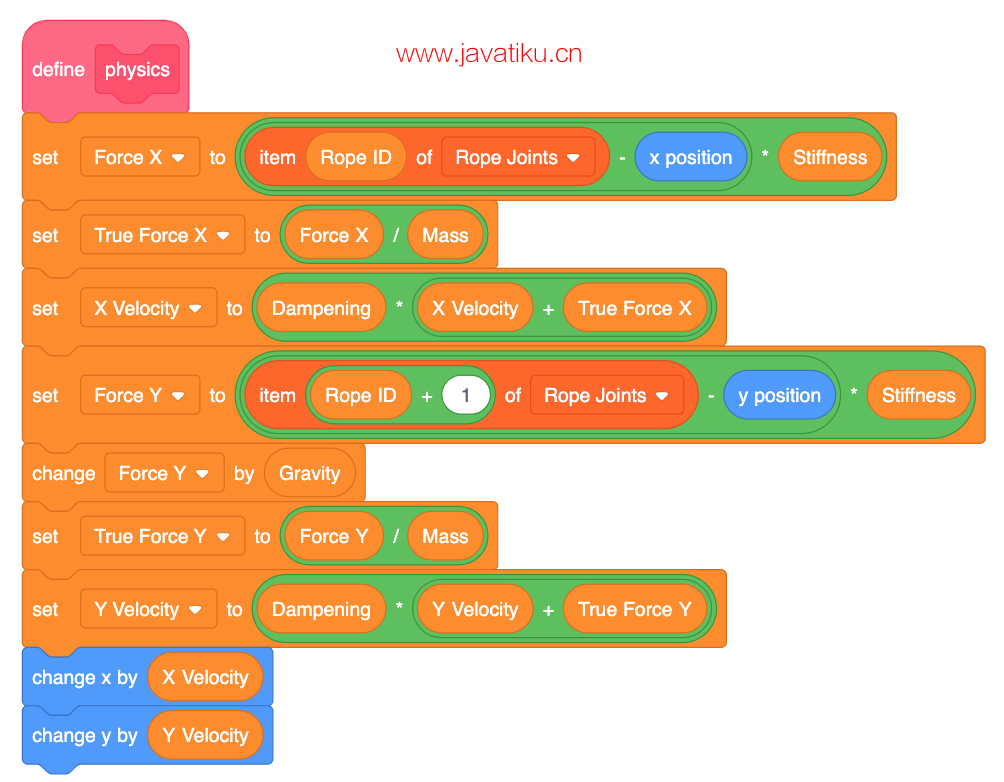## 摆锤弹簧绳索

### 变量和列表

• X速度
• Y速度

• 绳接头

• 制作的绳接头
• 关节ID

• 段长度

• ChainCreateList

• 删除的绳接头

• 关节类型

• 重力

• 距离
• X轴上的差异
• Y轴上的差异
• 绳张力
• X力
• Y力
• i
• 第二个关节点ID

• 玩家X速度
• 玩家Y速度

### 一些背景信息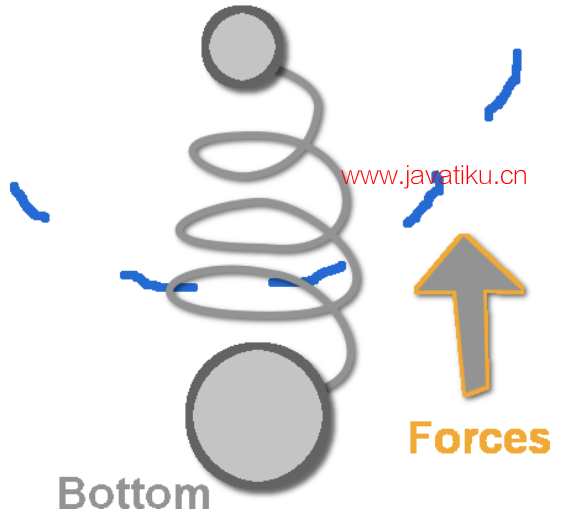Difference in X = x2 - x1

Difference in Y = y2 - y1

Distance = √((Difference in X)^2 + (Difference in Y)^2)

Rope Tension = (((Distance) - (Segment Length))/(Distance))

ForceX = ((-1) ((Rope Tension) (Difference in X)))

ForceY = ((-1) ((Rope Tension) (Difference in Y)))

### 编码

ChainCreateList

• 1 - 具有上方和下方绳索连接点的绳索连接点。
• 0 - 连接到对象的绳索连接点。对于本教程，它将连接到玩家角色。
• -1 - 仅连接到另一个绳索连接点的绳索连接点。

1, 1, 1, 0

• Rope Joint 1 的 X 位置
• Rope Joint 1 的 Y 位置
• Rope Joint 1 的类型
• Rope Joint 2 的 X 位置
• Rope Joint 2 的 Y 位置
• Rope Joint 2 的类型
• 以此类推。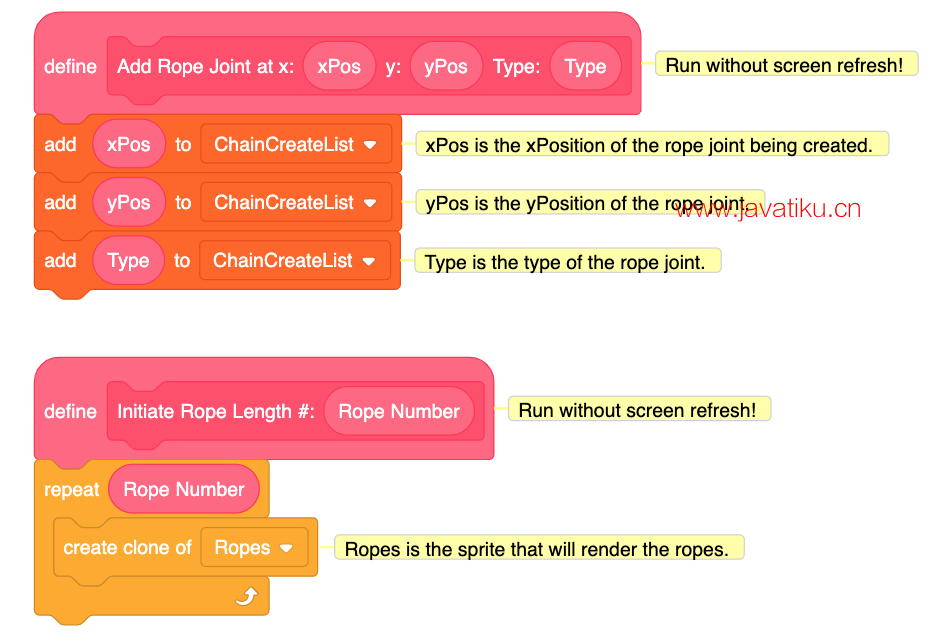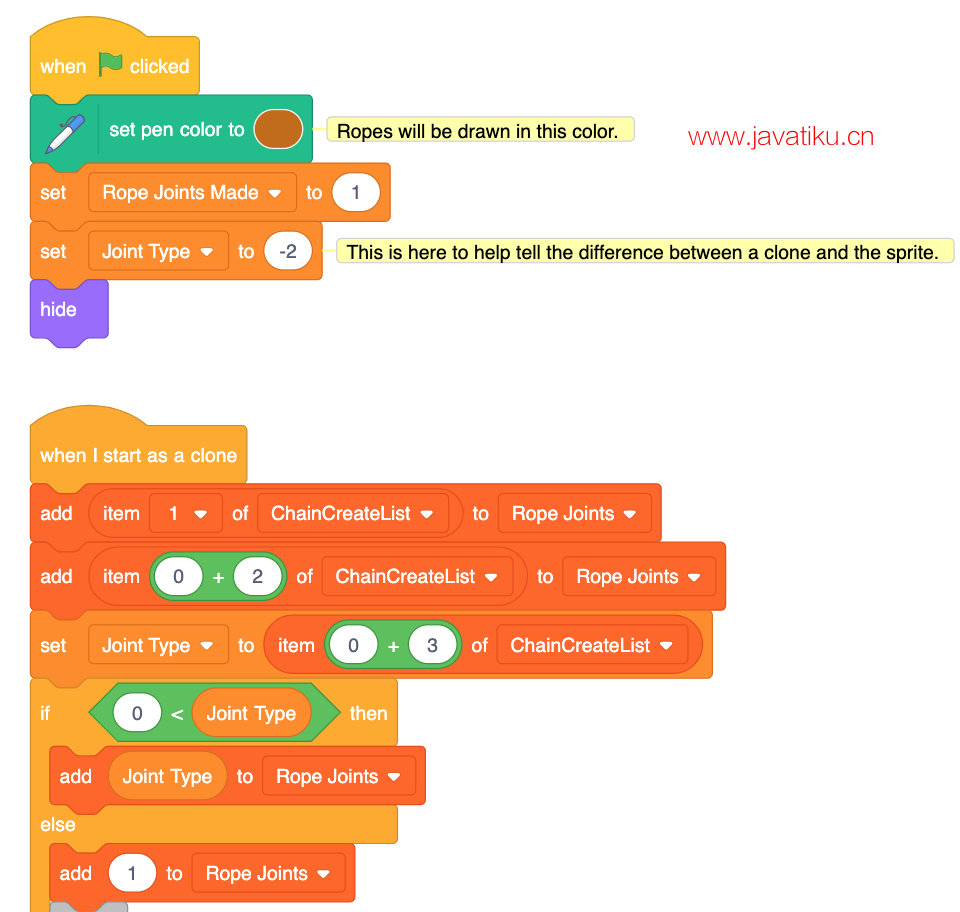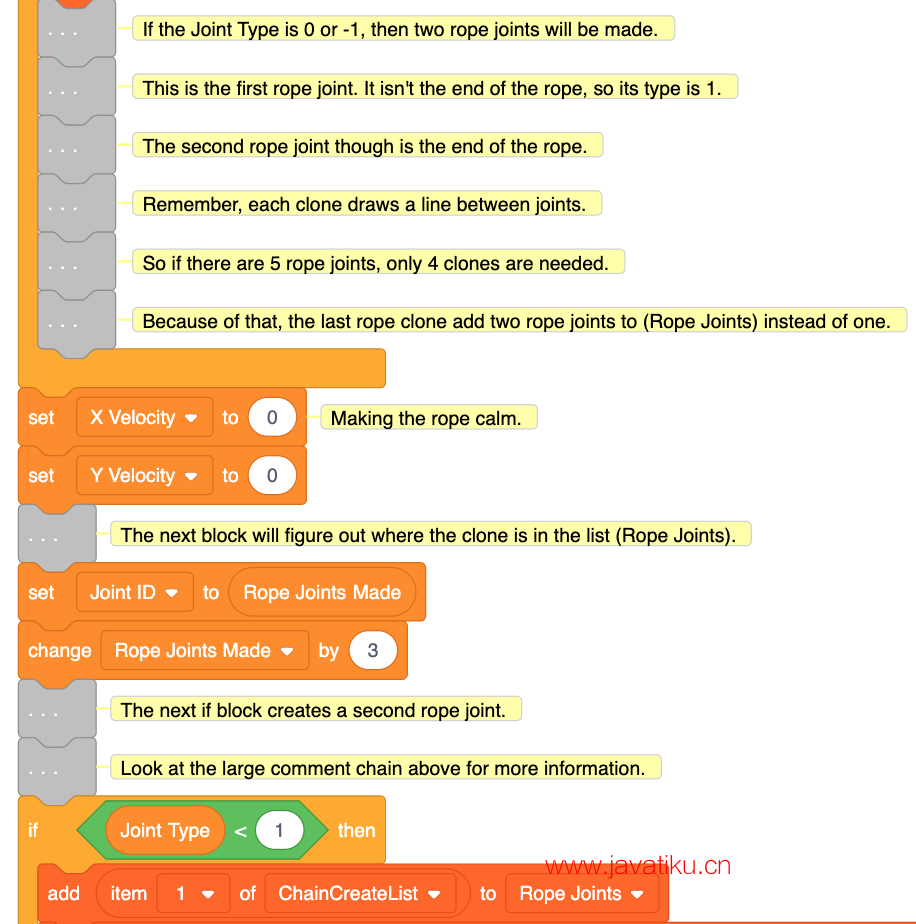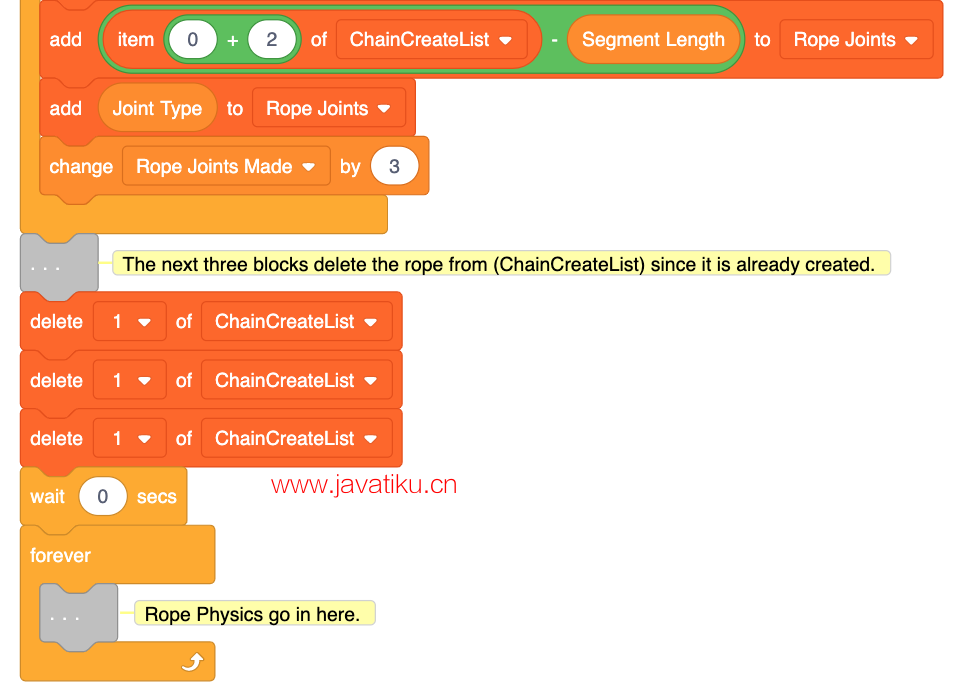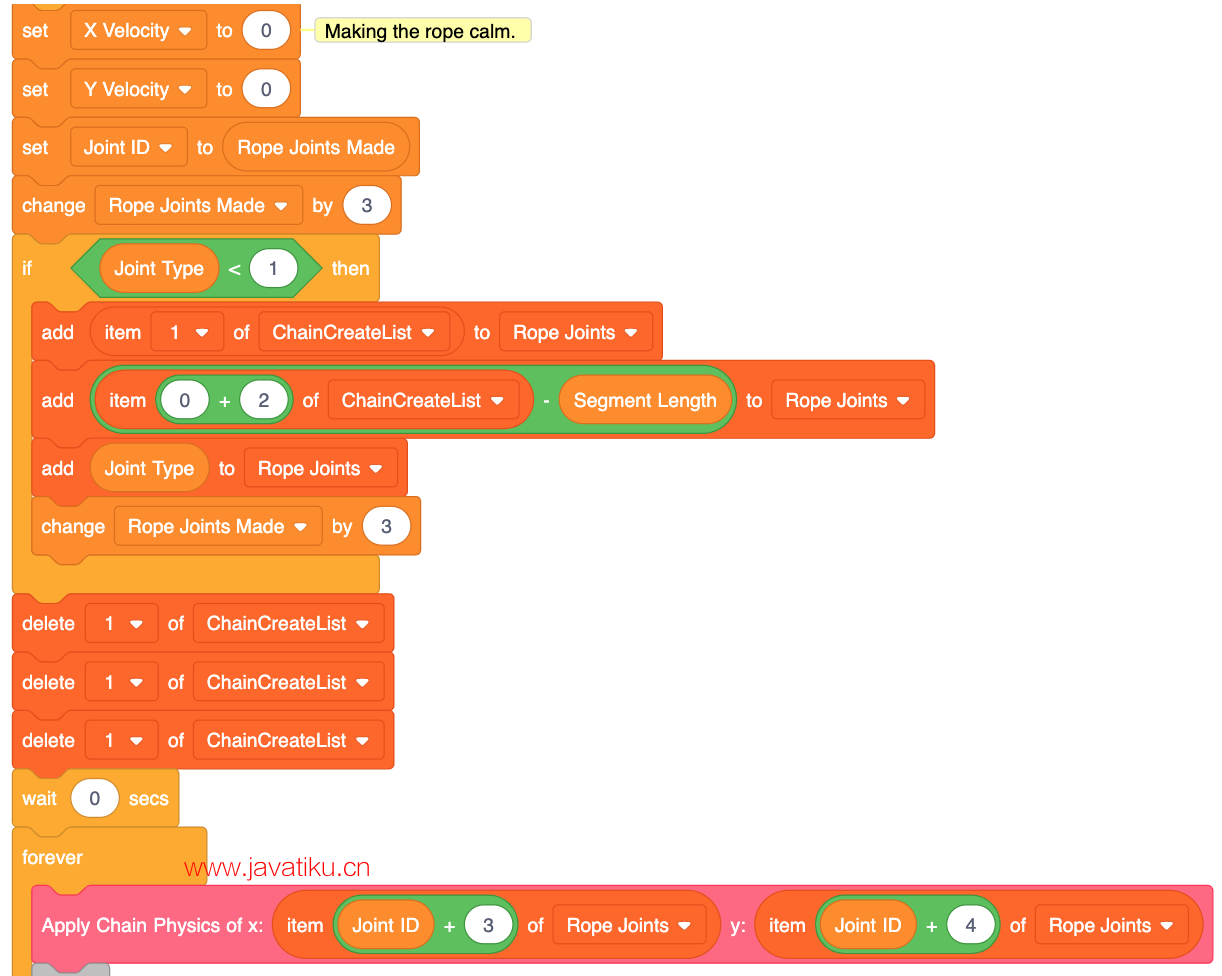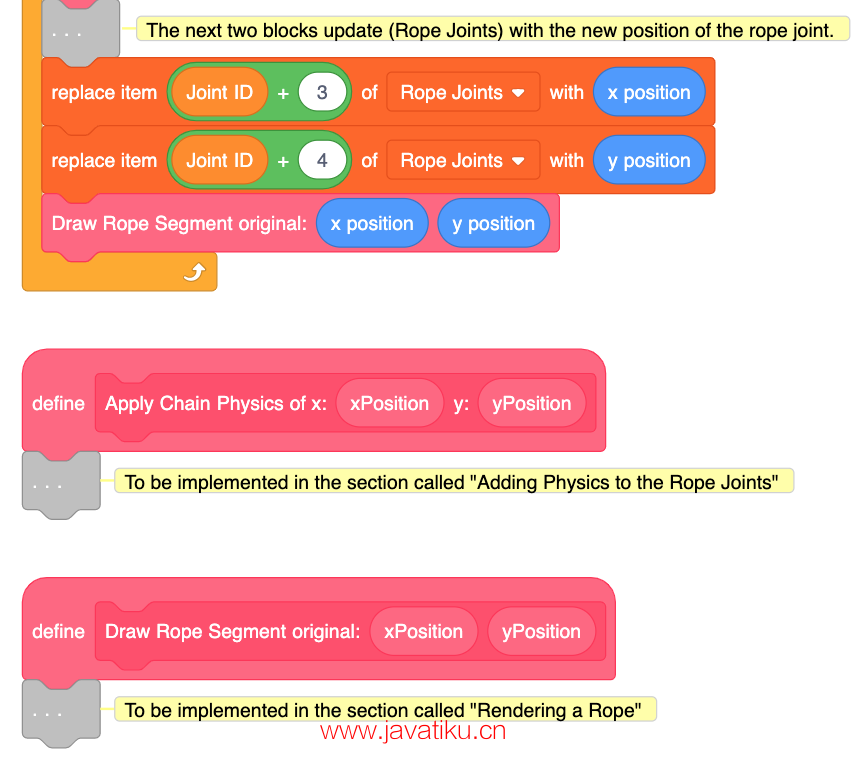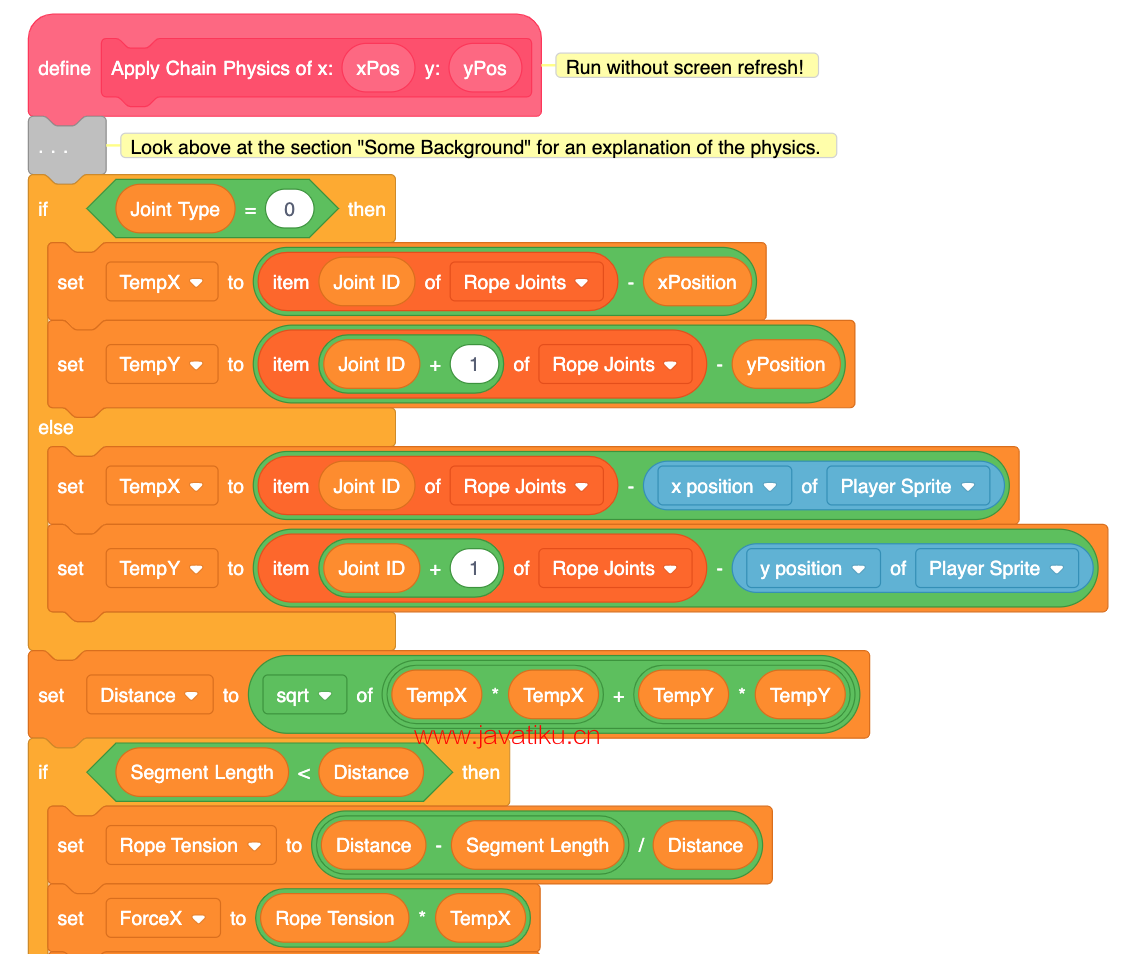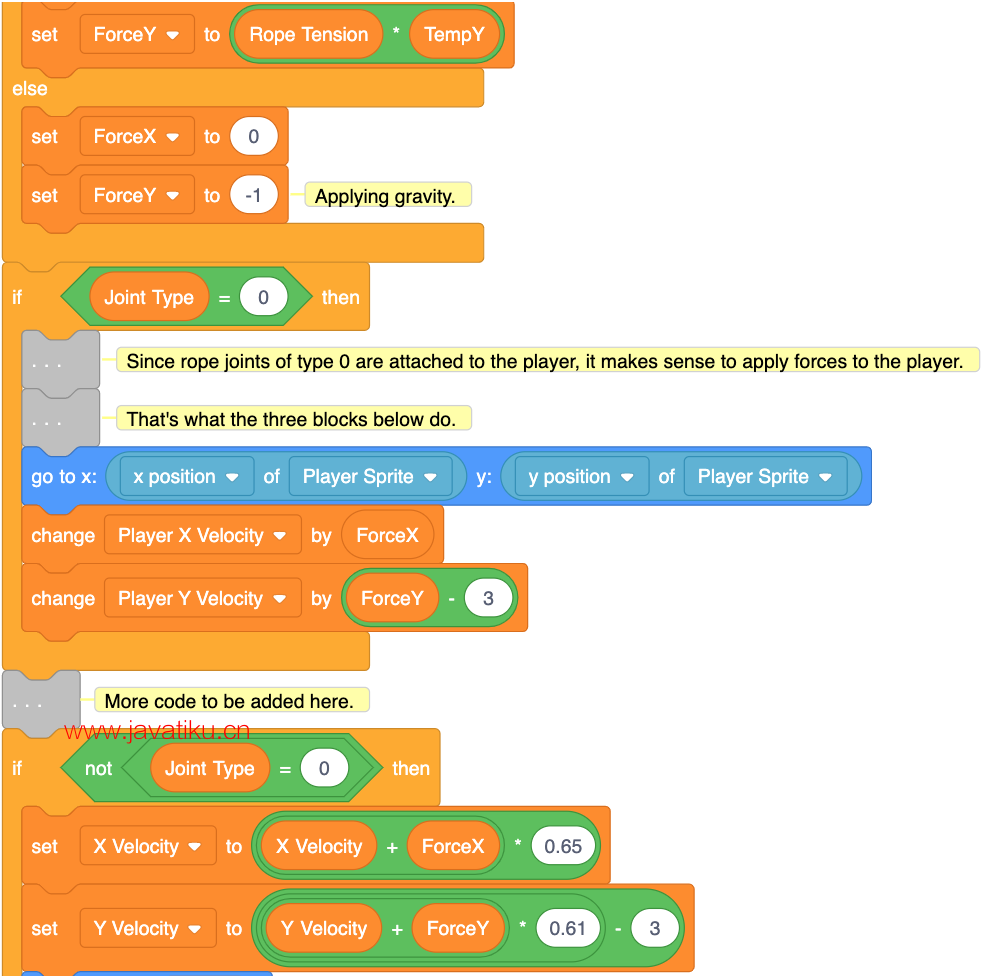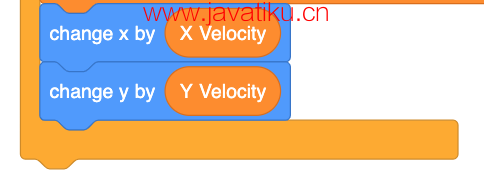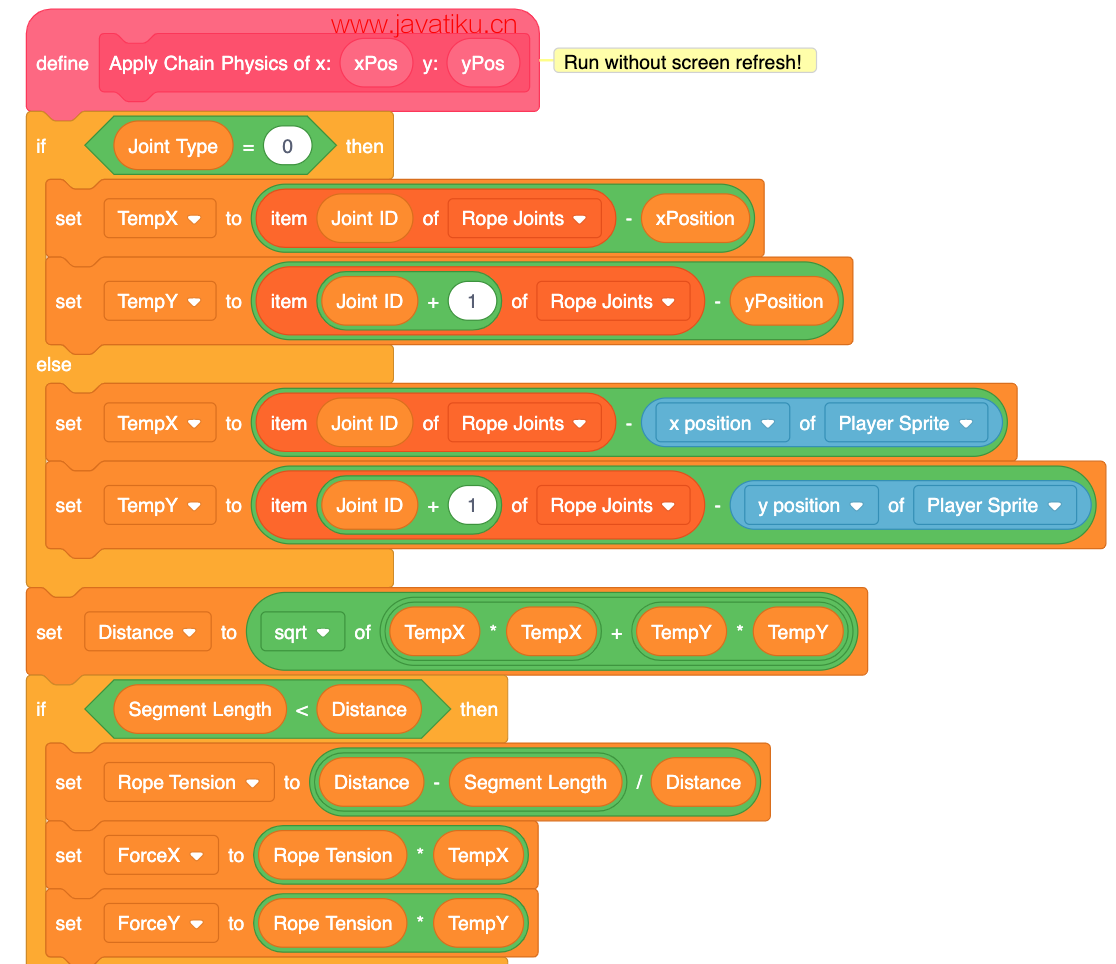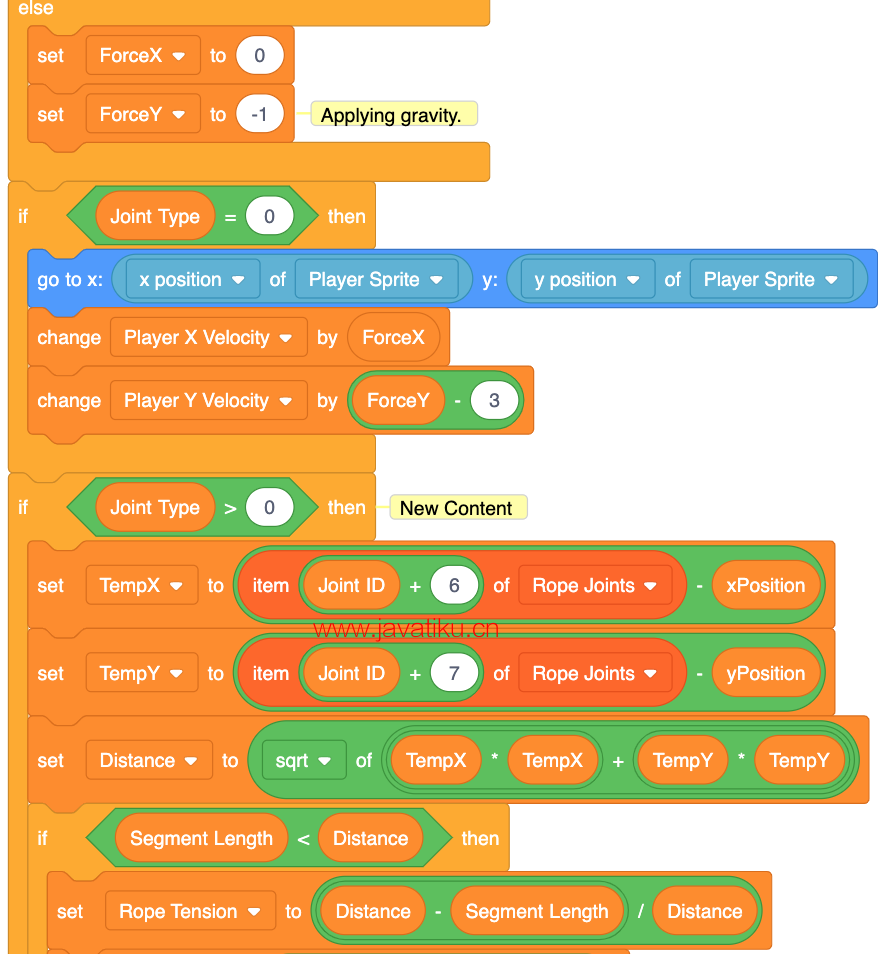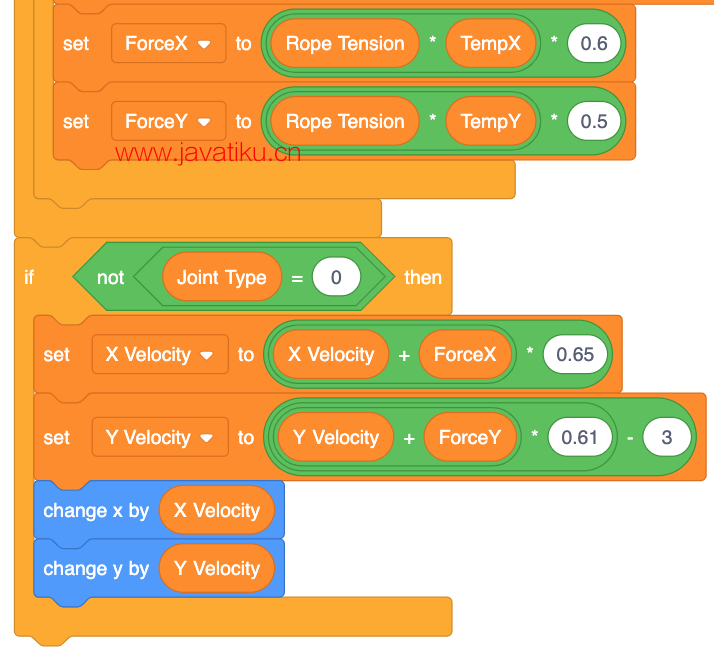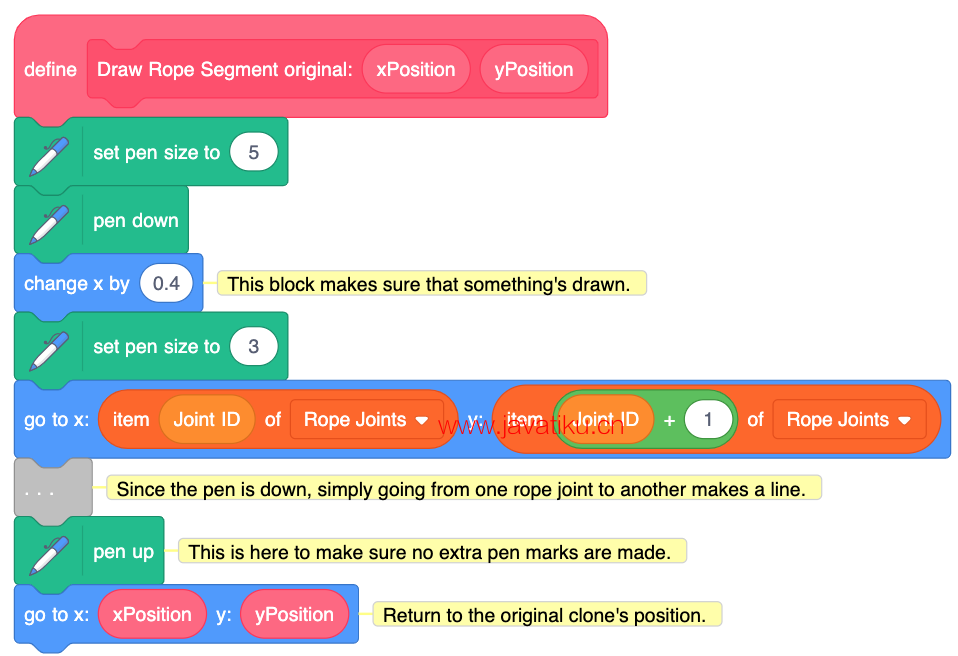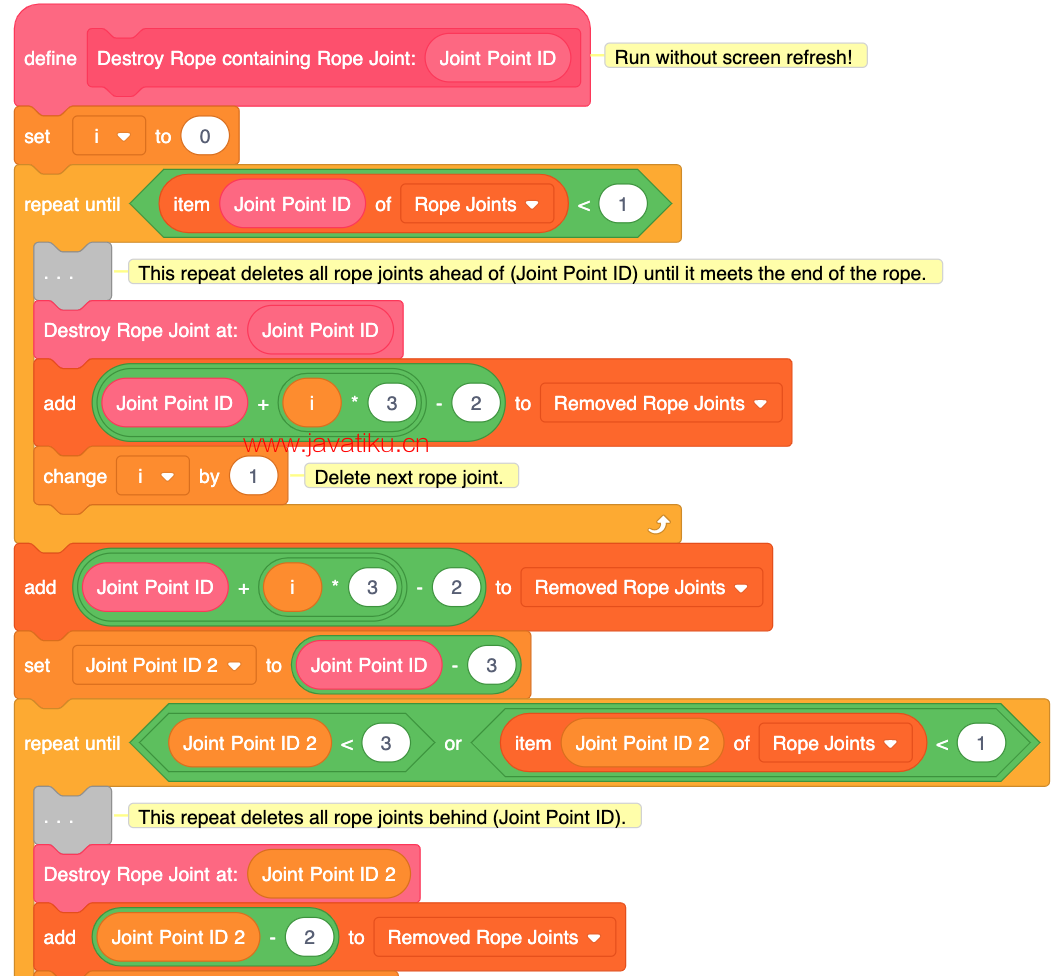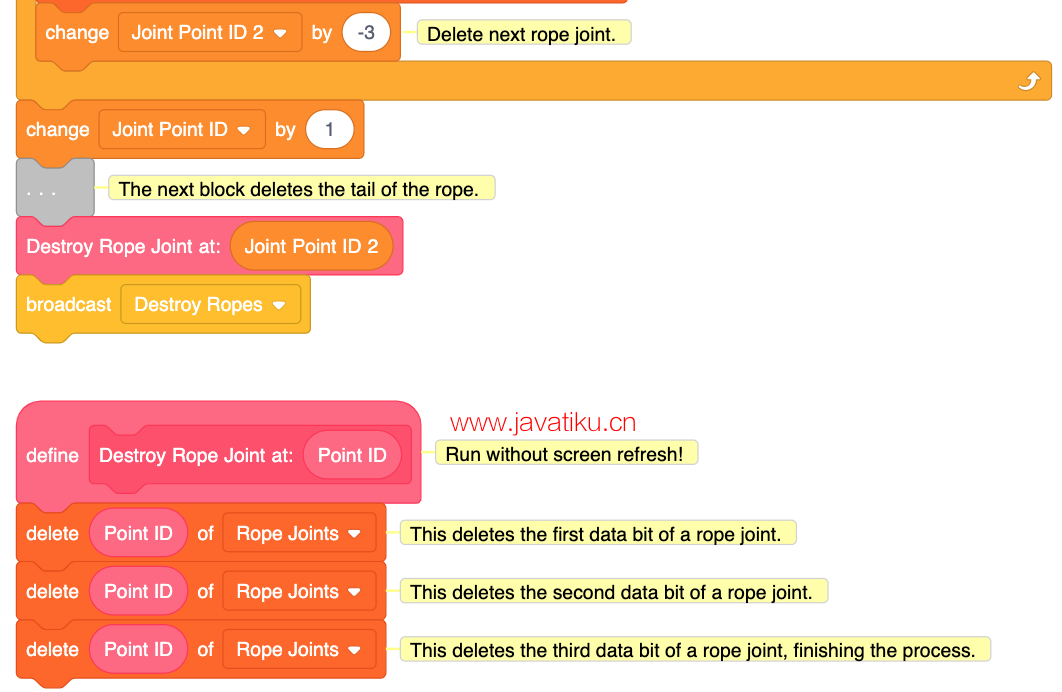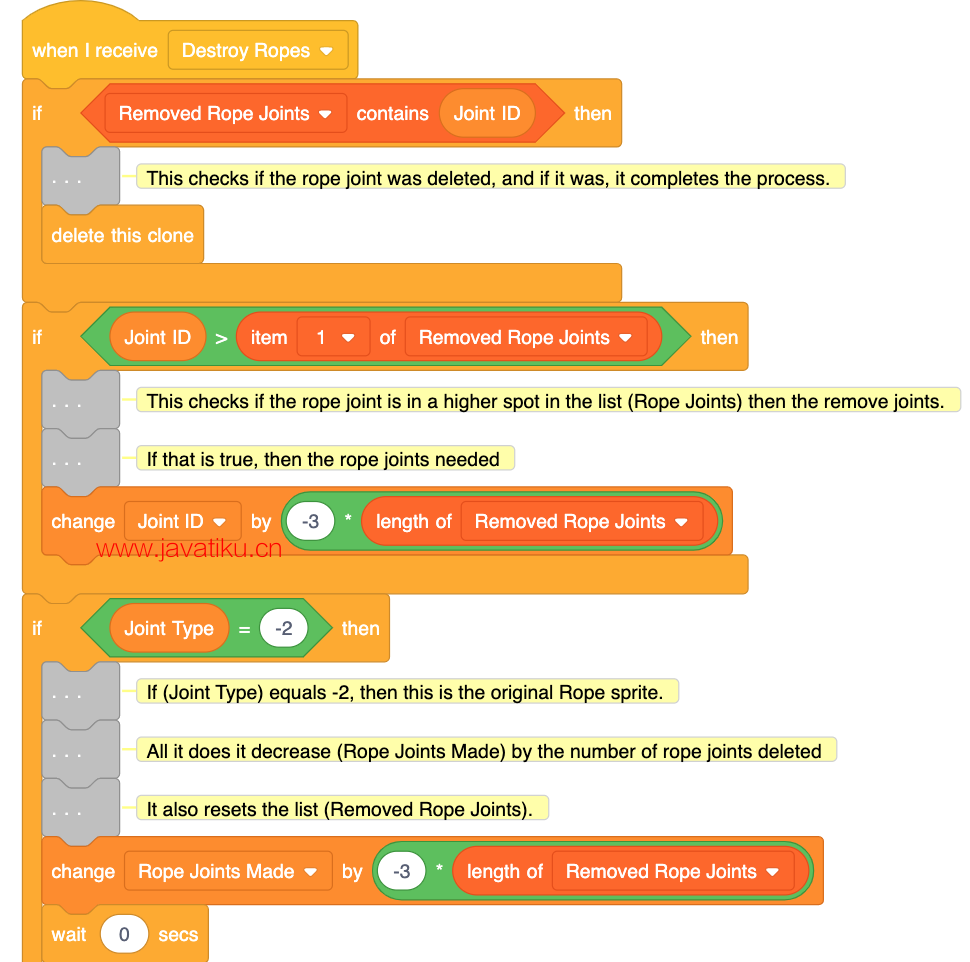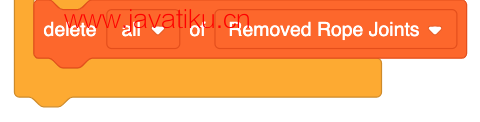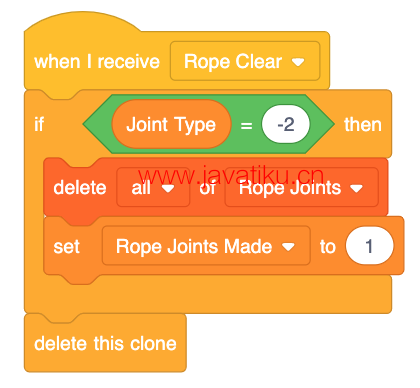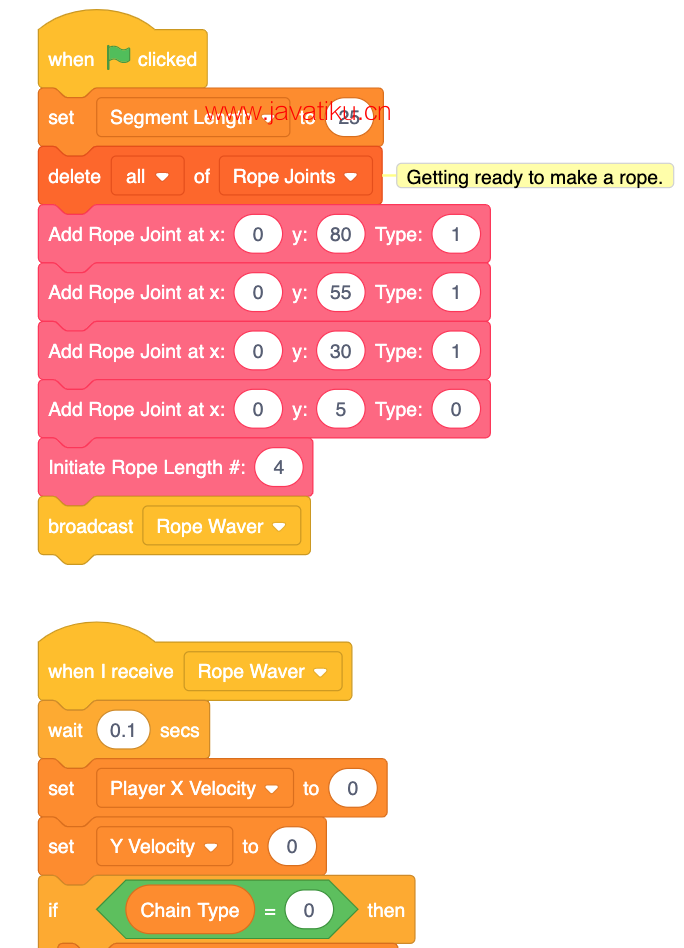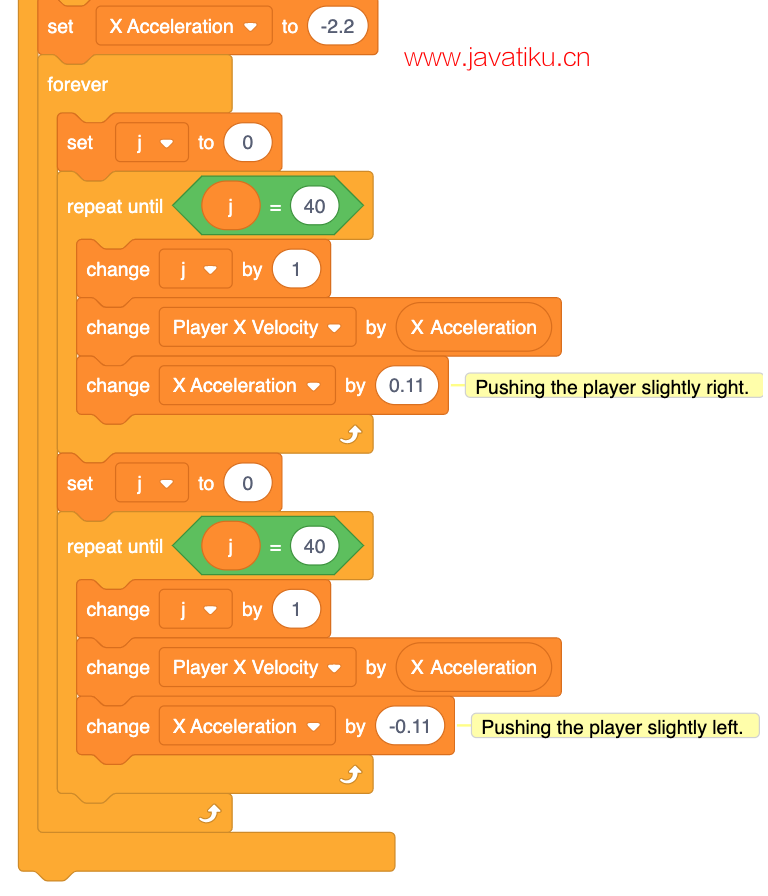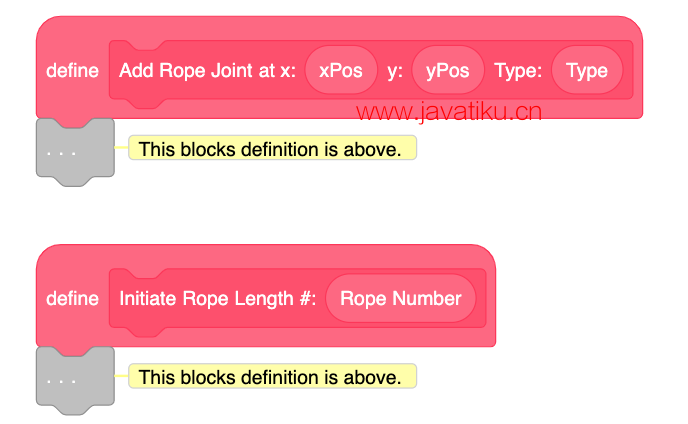• 重力
• 一个脚本来通过（Player X Velocity）改变x值
• 一个脚本来通过（Player Y Velocity）改变y值
• 一个脚本来减小（Player X Velocity）因为摩擦力。将（Player X Velocity）乘以0.65很好。
• 一个脚本来减小（Player Y Velocity）因为摩擦力。将（Player Y Velocity）乘以0.65很好。